Introduction

ggplot2.barplot is a function, to plot easily bar graphs using R software and ggplot2 plotting methods. This function is from easyGgplot2 package. An R script is available in the next section to install the package.

The aim of this tutorial is to show you step by step, how to plot and customize a bar chart using ggplot2.barplot function.

At the end of this document you will be able to draw, with few R code, the following plots :ggplot2.barplot function is described in detail at the end of this document.

easyGgplot2 R package can be installed as follow :

install.packages("devtools")
library(devtools)
install_github("easyGgplot2", "kassambara")

Load the package using this R code :

library(easyGgplot2)

Data format

The data must be a numeric vector or a data.frame (columns are variables and rows are observations).

restaurant data from easyGgplot2 package will be used in the following example.

#data.frame
##      sex   time total_bill
## 1 Female  Lunch      13.53
## 2 Female Dinner      16.81
## 3   Male  Lunch      16.24
## 4   Male Dinner      17.42

Basic barplot

A subset of restaurant data is used :

df<-restaurant[c(1,4), c("time", "total_bill")]
##     time total_bill
## 1  Lunch      13.53
## 4 Dinner      17.42
# Basic barplot plot of the 2 values of "total_bill" variables
ggplot2.barplot(data=df, xName="time", yName='total_bill')
# Change the width of bars
ggplot2.barplot(data=df, xName="time", yName='total_bill',
width=0.5)
# Change the orientation:Horizontal barplot plot
ggplot2.barplot(data=df, xName="time", yName='total_bill',
orientation="horizontal")
# y Axis reversed
ggplot2.barplot(data=df, xName="time", yName='total_bill',
orientation="yAxisReversed")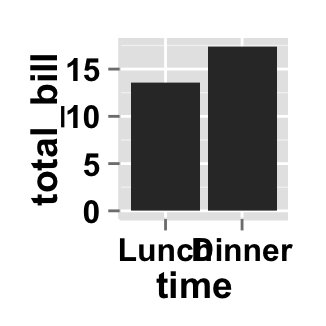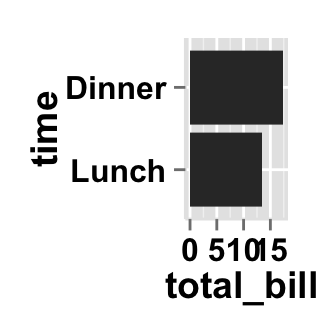Change the barplot line type and point shape

Different point shapes and line types can be used in the plot. By default, ggplot2 uses solid line type and circle shape.

ggplot2.barplot(data=df, xName="time", yName='total_bill',
# Change fill color and line color
fill="white", color="darkgreen",
# Change line type and line size
linetype="dashed", size=1.5)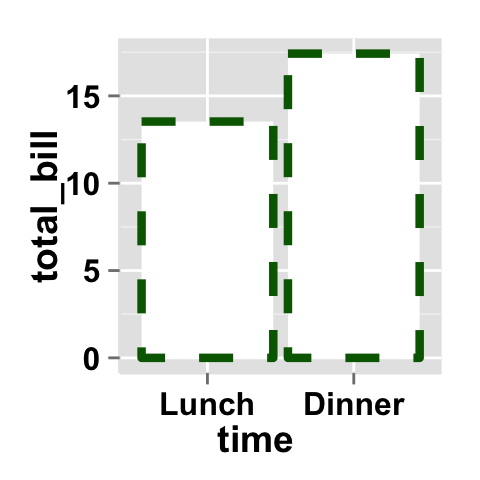Barplot of counts

In these examples, the height of the bar will represent the count of cases. To get a bar graph of counts, don’t map a variable to yName.

We’ll use the mtcars data :

# Data
##                    mpg cyl disp  hp drat    wt  qsec vs am gear carb
## Mazda RX4         21.0   6  160 110 3.90 2.620 16.46  0  1    4    4
## Mazda RX4 Wag     21.0   6  160 110 3.90 2.875 17.02  0  1    4    4
## Datsun 710        22.8   4  108  93 3.85 2.320 18.61  1  1    4    1
## Hornet 4 Drive    21.4   6  258 110 3.08 3.215 19.44  1  0    3    1
## Hornet Sportabout 18.7   8  360 175 3.15 3.440 17.02  0  0    3    2
## Valiant           18.1   6  225 105 2.76 3.460 20.22  1  0    3    1
# Barplot of counts. cyl(for the number of cylenders) is a factor.
# To get a bar graph of counts, don't map a variable to yName.
ggplot2.barplot(data=mtcars, xName="cyl")Parameters

The arguments that can be used to customize x and y axis are listed below :

Parameters Description
mainTitle the title of the plot
mainTitleFont a vector of length 3 indicating respectively the size, the style (“italic”, “bold”, “bold.italic”) and the color of x and y axis titles. Default value is: mainTitleFont=c(14, “bold”, “black”).
xShowTitle, yShowTitle if TRUE, x and y axis titles will be shown. Set the value to FALSE to hide axis labels. Default values are TRUE.
xtitle, ytitle x and y axis labels. Default values are NULL.
xtitleFont, ytitleFont a vector of length 3 indicating respectively the size, the style and the color of x and y axis titles. Possible values for the style:“plain”, “italic”, “bold”, “bold.italic”. Color can be specified as an hexadecimal code (e.g: “#FFCC00”) or by the name (e.g : “red”, “green”). Default values are xtitleFont=c(14,"bold", "black"), ytitleFont=c(14,"bold", "black").
xlim, ylim limit for the x and y axis. Default values are NULL.
xScale, yScale x and y axis scales. Possible values : c(“none”, “log2”, “log10”). e.g: yScale=“log2”. Default values are NULL.
xShowTickLabel, yShowTickLabel if TRUE, x and y axis tick mark labels will be shown. Default values are TRUE.
xTickLabelFont, yTickLabelFont a vector of length 3 indicating respectively the size, the style and the color of x and y axis tick label fonts. Default value are xTickLabelFont=c(12, "bold", "black"), yTickLabelFont=c(12, "bold", "black").
xtickLabelRotation, ytickLabelRotation Rotation angle of x and y axis tick labels. Default value are 0.
hideAxisTicks if TRUE, x and y axis ticks are hidden. Default value is FALSE.
axisLine a vector of length 3 indicating respectively the size, the line type and the color of axis lines. Default value is c(0.5, "solid", "#E5E5E5").

Main title and axis labels

# Change main title and axis titles
ggplot2.barplot(data=df, xName="time", yName='total_bill',
mainTitle="Total bill\n per time of day",
xtitle="Time of day", ytitle="Total bill")
# Customize title styles. Possible values for the font style :
# 'plain', 'italic', 'bold', 'bold.italic'.
ggplot2.barplot(data=df, xName="time", yName='total_bill',
mainTitle="Plot of total bill\n per time of day",
xtitle="Time of day", ytitle="Total bill",
mainTitleFont=c(14,"bold.italic", "red"),
xtitleFont=c(14,"bold", "#993333"),
ytitleFont=c(14,"bold", "#993333"))
# Hide x an y axis titles
ggplot2.barplot(data=df, xName="time", yName='total_bill',
xShowTitle=FALSE, yShowTitle=FALSE)Axis ticks

# Axis ticks labels and orientaion
ggplot2.barplot(data=df, xName="time", yName='total_bill',
xShowTitle=FALSE, yShowTitle=FALSE,
xTickLabelFont=c(14,"bold", "#993333"),
yTickLabelFont=c(14,"bold", "#993333"),
xtickLabelRotation=45, ytickLabelRotation=45)
#Hide axis tick labels
ggplot2.barplot(data=df, xName="time", yName='total_bill',
xShowTitle=FALSE, yShowTitle=FALSE,
xShowTickLabel=FALSE, yShowTickLabel=FALSE)
#Hide axis ticks
ggplot2.barplot(data=df, xName="time", yName='total_bill',
xShowTitle=FALSE, yShowTitle=FALSE,
xShowTickLabel=FALSE, yShowTickLabel=FALSE,
hideAxisTicks=TRUE)
# AxisLine : a vector of length 3 indicating the size,
#the line type and the color of axis lines
ggplot2.barplot(data=df, xName="time", yName='total_bill',
axisLine=c(1, "solid", "darkblue"))Background and colors

Change barplot background and fill colors

# change background color to "white". Default is "gray"
ggplot2.barplot(data=df, xName="time", yName='total_bill',
backgroundColor="white")
# Change background color to "lightblue" and grid color to "white"
ggplot2.barplot(data=df, xName="time", yName='total_bill',
backgroundColor="lightblue", gridColor="white")
# Change plot fill color
ggplot2.barplot(data=df, xName="time", yName='total_bill',
color="black")
# Remove grid; Remove Top and right border around the plot
ggplot2.barplot(data=df, xName="time", yName='total_bill',
removePanelGrid=TRUE,removePanelBorder=TRUE,
axisLine=c(0.5, "solid", "black"))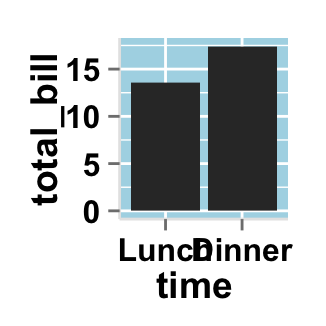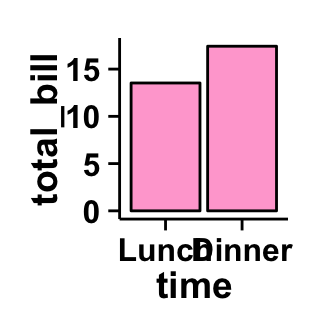Change barplot color according to the group

Colors can be specified as a hexadecimal RGB triplet, such as "#FFCC00" or by names (e.g : "red" ). You can also use other color scales, such as ones taken from the RColorBrewer package. The different color systems available in R have been described in detail here.

To change barplot color according to the group, you have to specify the name of the data column containing the groups using the argument groupName. Use the argument groupColors, to specify colors by hexadecimal code or by name. In this case, the length of groupColors should be the same as the number of the groups. Use the argument brewerPalette, to specify colors using RColorBrewerpalette.

# Color the barplot by groupName
ggplot2.barplot(data=df, xName="time", yName='total_bill',
groupName="time")
# Change group colors using hexadecimal colors;
ggplot2.barplot(data=df, xName="time", yName='total_bill',
groupName="time",
#background and line colors
backgroundColor="white", color="black",
groupColors=c('#999999','#E69F00'))

# Change group colors using brewer palette: "Paired"
ggplot2.barplot(data=df, xName="time", yName='total_bill',
groupName="time",
#background and line colors
backgroundColor="white", color="black",
brewerPalette="Paired")
# Change group colors using color names
ggplot2.barplot(data=df, xName="time", yName='total_bill',
groupName="time",
#background and line colors
backgroundColor="white", color="black",
groupColors=c('aquamarine3','chartreuse1'))Legend

Legend position

# Change the legend position to "top"
# (possible values: "left","top", "right", "bottom")
ggplot2.barplot(data=df, xName="time", yName='total_bill',
groupName="time", legendPosition="top")
# legendPosition can be also a numeric vector c(x, y)
ggplot2.barplot(data=df, xName="time", yName='total_bill',
groupName="time", legendPosition=c(0.8,0.2))
# Remove plot legend
ggplot2.barplot(data=df, xName="time", yName='total_bill',
groupName="time",showLegend=FALSE)It is also possible to position the legend inside the plotting area. You have to indicate the x, y coordinates of legend box. x and y values must be between 0 and 1. c(0,0) corresponds to “bottom left” and c(1,1) corresponds to “top right” position.

Legend background color, title and text font styles

# Change legend background color, title and text font styles
ggplot2.barplot(data=df, xName="time", yName='total_bill',
groupName="time",
# legendPosition=c("right", "left","top", "bottom")
legendPosition="right",
# legendTitleFont=c(size, style, color)
legendTitle="Time", legendTitleFont=c(10, "bold", "blue"),
# legendTextFont=c(size, style, color)
legendTextFont=c(10, "bold.italic", "red"),
# legendBackground: c(fill, lineSize, lineType, lineColor)
legendBackground=c("lightblue", 0.5, "solid", "darkblue" )
)Axis scales

Possible values for y axis scale are “none”, “log2” and log10. Default value is “none”.

# Change y axis limit
ggplot2.barplot(data=df, xName="time", yName='total_bill',
ylim=c(0,50))

# y Log scale. yScale="log2".
# Possible value="none", "log2" and "log10"
ggplot2.barplot(data=df, xName="time", yName='total_bill',
yScale="log2")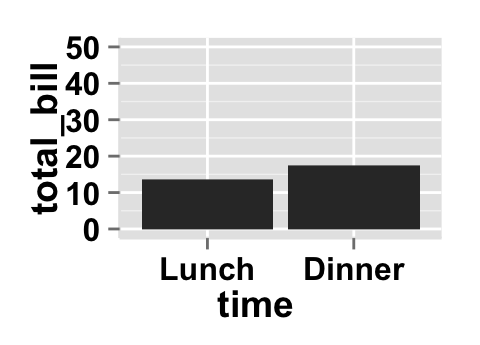Create a customized plots with few R code

# Customized barplot
ggplot2.barplot(data=df, xName="time", yName='total_bill',
groupName="time",
groupColors=c('#999999','#E69F00'), showLegend=FALSE,
backgroundColor="white", xtitle="Time of day",
ytitle="Total bill",
mainTitle="Total bill per \n time of day")
# Remove grid; Remove Top and right border around the plot
ggplot2.barplot(data=df, xName="time", yName='total_bill',
groupName="time",
groupColors=c('#999999','#E69F00'), showLegend=FALSE,
backgroundColor="white", color="black",
xtitle="Time of day", ytitle="Total bill",
mainTitle="Total bill per \n time of day",
removePanelGrid=TRUE,removePanelBorder=TRUE,
axisLine=c(0.5, "solid", "black"))Barplot with multiple groups

This data will be used for the examples below:

df1 <- restaurant
##      sex   time total_bill
## 1 Female  Lunch      13.53
## 2 Female Dinner      16.81
## 3   Male  Lunch      16.24
## 4   Male Dinner      17.42
# Stacked barplot
# Plot of variable 'total_bill' according to xName 'time'.
# The plot is colored by the groupName 'sex'
ggplot2.barplot(data=df1, xName='time', yName="total_bill",
groupName='sex')
# Use position=position_dodge()
ggplot2.barplot(data=df1, xName='time', yName="total_bill",
groupName='sex', position=position_dodge())
# change colors
ggplot2.barplot(data=df1, xName='time', yName="total_bill",
groupName='sex', groupColors=c('#999999','#E69F00'),
position=position_dodge(),
#background and line colors
backgroundColor="white", color="black",
xtitle="Time of day", ytitle="Total bill",
mainTitle="Total bill\n per time of day",
removePanelGrid=TRUE,removePanelBorder=TRUE,
axisLine=c(0.5, "solid", "black")
)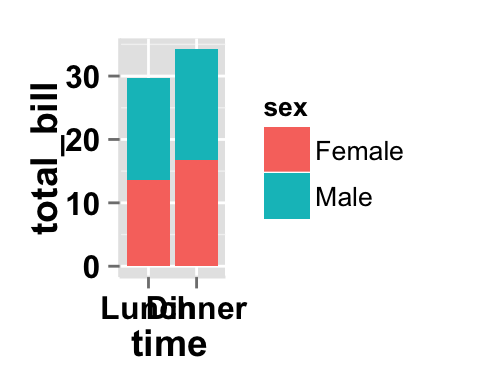In the following examples, diamonds dataset from ggplot2 package is used.

##   carat       cut color clarity depth table price    x    y    z
## 1  0.23     Ideal     E     SI2  61.5    55   326 3.95 3.98 2.43
## 2  0.21   Premium     E     SI1  59.8    61   326 3.89 3.84 2.31
## 3  0.23      Good     E     VS1  56.9    65   327 4.05 4.07 2.31
## 4  0.29   Premium     I     VS2  62.4    58   334 4.20 4.23 2.63
## 5  0.31      Good     J     SI2  63.3    58   335 4.34 4.35 2.75
## 6  0.24 Very Good     J    VVS2  62.8    57   336 3.94 3.96 2.48
# stacked bar chart; Barplot of the count
ggplot2.barplot(data=diamonds, xName="clarity",
groupName="cut")
# change the color
ggplot2.barplot(data=diamonds, xName="clarity",
groupName="cut", brewerPalette="Blues")Faceting : split a plot into a matrix of pannels

The facet approach splits a plot into a matrix of panels. Each panel shows a different subset of the data.

Facet with one variable

# Facet according to the cut variable
ggplot2.barplot(data=diamonds, xName="clarity",
faceting=TRUE, facetingVarNames="cut")
# Change the direction.
# possible values are "vertical", "horizontal".
# default is vertical.
ggplot2.barplot(data=diamonds, xName="clarity",
faceting=TRUE, facetingVarNames="cut",
facetingDirection="horizontal")Facet with two variables

# Facet by two variables: cut and color.
# Rows are cut and columns are color
ggplot2.barplot(data=diamonds, xName="clarity",
faceting=TRUE,
facetingVarNames=c("cut", "color"))
# Facet by two variables: reverse the order of the 2 variables
# Rows are color and columns are cut
ggplot2.barplot(data=diamonds, xName="clarity",
faceting=TRUE, facetingVarNames=c( "color", "cut"))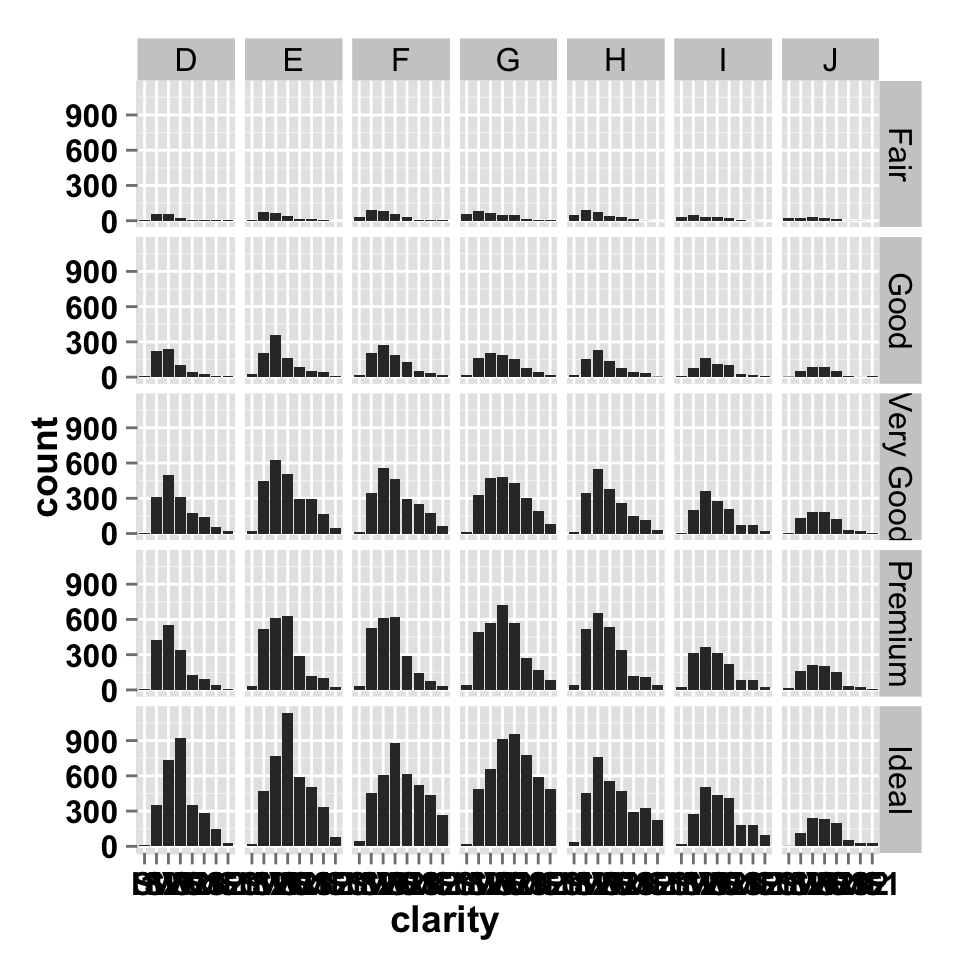Facet scales

By default, all the panels have the same scale (facetingScales="fixed"). They can be made independent, by setting scales to free, free_x, or free_y.

# Facet with free scales
ggplot2.barplot(data=diamonds, xName="clarity",
faceting=TRUE, facetingVarNames=c("cut", "color"),
facetingScales="free")As you can see in the above plot, y axis have different scales in the different panels.

Facet label apperance

# Change facet text font
# Possible values for the font style:'plain', 'italic', 'bold', 'bold.italic'.
ggplot2.barplot(data=diamonds, xName="clarity",
faceting=TRUE, facetingVarNames=c("cut", "color"),
facetingFont=c(12, 'bold.italic', "red"))
#Change the apperance of the rectangle around facet label
ggplot2.barplot(data=diamonds, xName="clarity",
faceting=TRUE, facetingVarNames=c("cut", "color"),
facetingRect=list(background="white", lineType="solid",
lineColor="black", lineSize=1.5)
)ggplot2.barplot function

Description

Plot easily a barplot using easyGgplot2 R package.

usage

ggplot2.barplot(data, xName=NULL, yName=NULL,
groupName=NULL,
groupColors=NULL, brewerPalette=NULL,...)

Arguments

Arguments Descriptions
data data.frame or a numeric vector. Columns are variables and rows are observations.
xName The name of column containing x variable (i.e groups). Default value is NULL.
yName The name of column containing y variable. If yName=NULL, data should be a numeric vector.
groupName The name of column containing group variable. This variable is used to color plot according to the group.
groupColors Color of groups. groupColors should have the same length as groups.
brewerPalette This can be also used to indicate group colors. In this case the parameter groupColors should be NULL. e.g: brewerPalette=“Paired”.
…. Other arguments passed on to ggplot2.customize custom function or to geom_bar functions from ggplot2 package.

The other arguments which can be used are described at this link : ggplot2 customize. They are used to customize the plot (axis, title, background, color, legend, ….) generated using ggplot2 or easyGgplot2 R package.

Examples

library(easyGgplot2)
#data
df <- restaurant[c(1,4), c("time", "total_bill")]
#plot
ggplot2.barplot(data=df, xName="time", yName='total_bill',
mainTitle="Total bill\n per time of day",
xtitle="Time of day", ytitle="Total bill")
# Or use this
plot<-ggplot2.barplot(data=df, xName="time", yName='total_bill')
plot<-ggplot2.customize(plot,
mainTitle="Total bill\n per time of day",
xtitle="Time of day", ytitle="Total bill")
print(plot)

Easy ggplot2 ebook

Note that an eBook is available on easyGgplot2 package here.

September 2014 : First edition.

Licence : This document is under creative commons licence (http://creativecommons.org/licenses/by-nc-sa/3.0/).

Infos

This analysis was performed using R (ver. 3.1.0), easyGgplot2 (ver 1.0.0) and ggplot2 (ver 1.0.0).